# Using PostGIS for isovists calculation

First of all, what is an `isovist`? It’s simply the same as a `viewshed`… and what the heck is a `viewshed`? Let’s go to the Wikipedia:

``````A single isovist is the volume of space visible from a given point in space,
together with a specification of the location of that point
``````

The decision of using the word `isovist` or `viewshed` is only related to the knowledge field you’re working on:

• Isovist: architecture, space syntax, landscaping, urbanism, geomarketing
• viewshed: geography, infrastructure location

In this article, I’m going to talk about the 2D version of the problem, but could be expanded to cover the 3D case.

Let’s define a real use case as a base for later explanations. Let’s say we’re and ad company and want to place billboards in the facades of buildings in the center of Madrid, targeting the people waiting for the bus at the defined stops. Our target would be to find the facades with the highest `eyeballs` audience. The result should be like this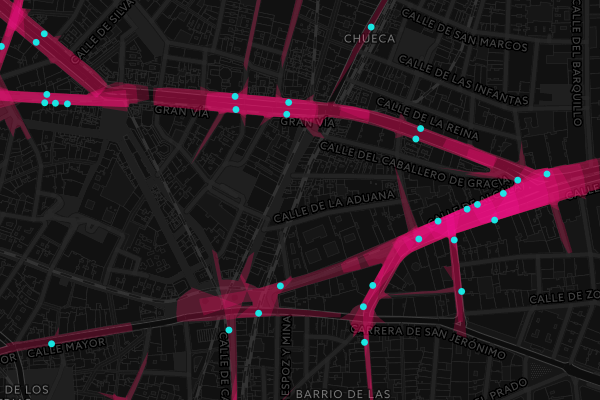Cool, isn’t it? And how is it done? The answer is quite simpleYes, magic.

And PostGIS.

Ok, follow me in this journey along this ray-tracing-like approach using a somehow tricky PL/pgSQL function

Let’s say, that the point I’m going to study (`center`) has a heading of 270º and a fov of 90º, and the horizon is located at 150m. The arbitrary point for the following images is defined as:

``````SELECT ST_SetSRID(ST_MakePoint( -3.705856,40.420371),4326) as center;
``````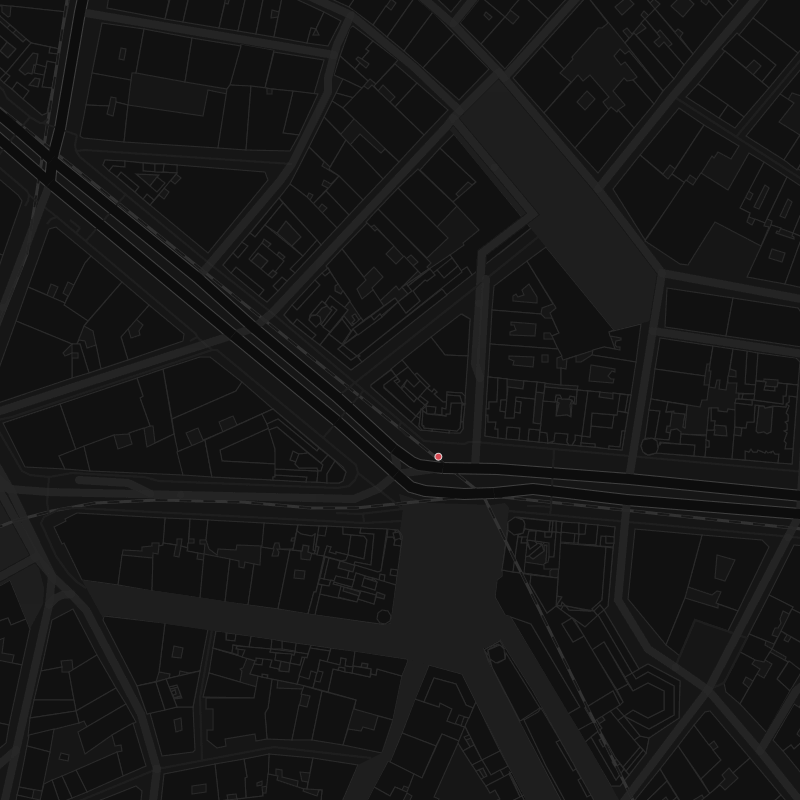First of all, let’s think on the macro structure of the problem. We will need a point geometry for any input point and a set of `buildings` polygons. But… we will need some extra parameters too, like:

• `radius`: Horizon distance, so we don’t overcharge the calculation with useless info
• `rays`: number of angular steps, that will define the resolution
• `heading`: northing in degrees, to set the direction the `eyeballs` are looking at
• `fov`: field of view in degrees, centered in the heading directionSo the signature of the function would be like:

``````CREATE OR REPLACE FUNCTION ISOVIST(
IN center geometry,
IN polygons geometry[],
IN radius numeric DEFAULT 150, -- horizon distance in meters
IN rays integer DEFAULT 36, -- number of rays
IN heading integer DEFAULT -999, -- take heading (degrees, 0-360) into account
IN fov integer DEFAULT 360 -- field of view (degrees)
)
``````

Having a default value for `fov` of 360º, so the heading is not needed in this very case.

Once there, let’s declare some support variables

``````    arc numeric; -- angular resolution
angle_0 numeric; -- starting angle for fov != 360º
``````

And the output

``````    geomout geometry; -- resulting geometry of the isovist
``````

And calc the values for the support variables:

``````    -- resolution in degrees
arc := fov::numeric / rays::numeric;

-- fov range start value
IF fov = 360 THEN
angle_0 := 0;
ELSE
angle_0 := heading - 0.5 * fov;
END IF;
``````

Now, we’re ready to start querying the data. We’re building it with incremental subqueries using `WITH` clause, so each block can be easily explained:

1. Unnest the polygons array to have a table object:

``````     buildings_0 AS(
SELECT
t.geom
FROM unnest(polygons) as t(geom)
),
``````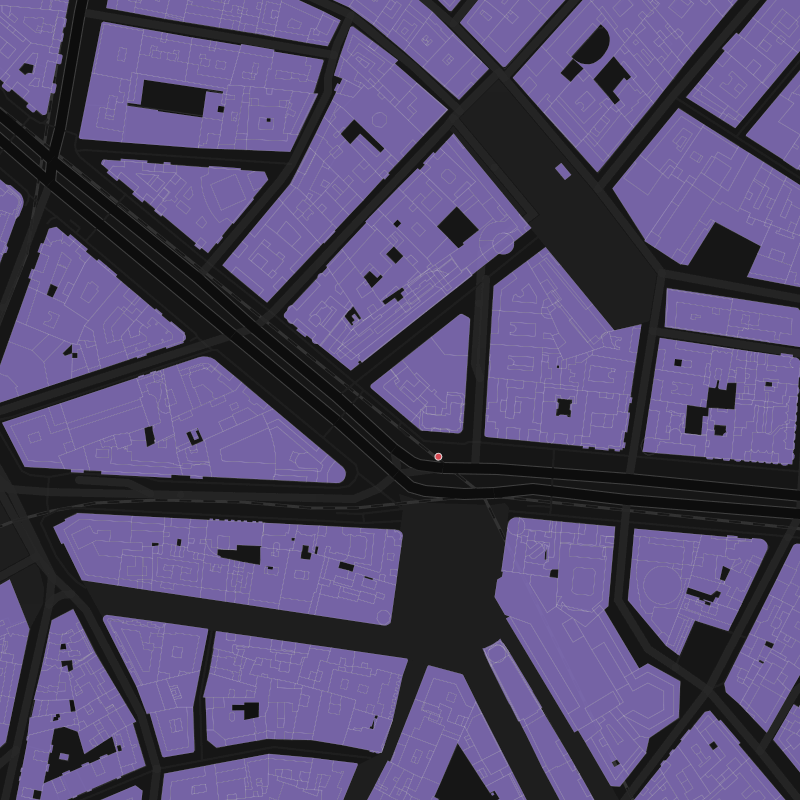2. Filter out the polygons further than the horizon, so we limit the next calculi to the polygons of interest only

``````   buildings_crop AS(
SELECT
geom
FROM buildings_0
WHERE
ST_DWithin(
center::geography,
geom::geography,
)
),
``````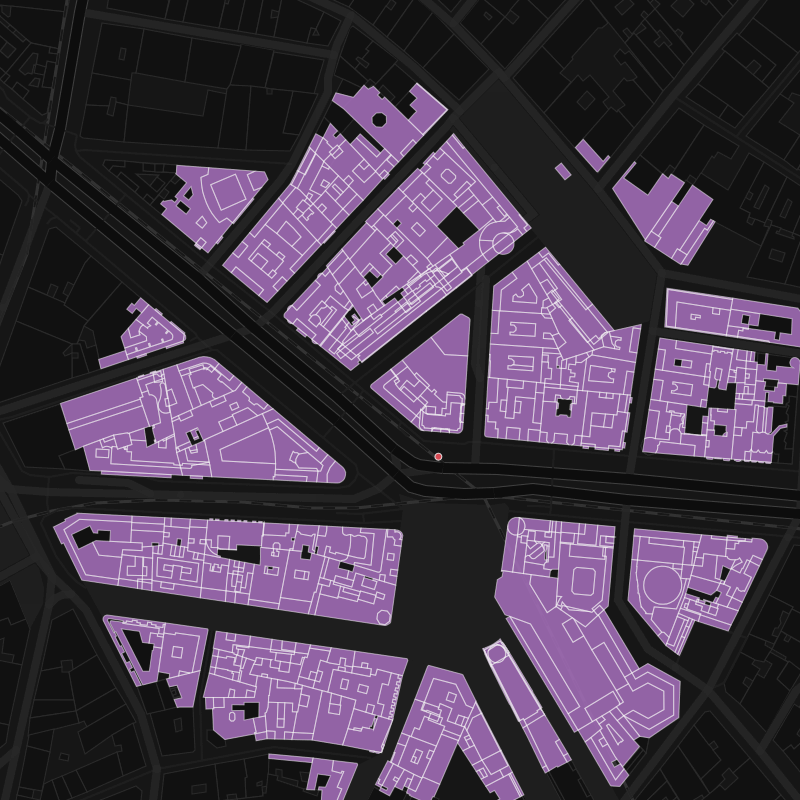3. Let’s add the horizon as `the final polygon` that stops any ray traced from `center`

``````    buildings AS(
SELECT geom FROM buildings_crop
UNION ALL
),
``````

4. Now, we need to create #`rays` rays, from `center`, each `arc` degrees starting at `angle_0` . To do so, we’re gonna use the PostGIS function ST_Project that generates a 2nd point from a starting one, a direction and a distance. So my n-th ray will look like

``````ST_SetSRID(
ST_MakeLine(
center,
ST_Project(
center::geography,
)::geometry
),
4326) AS geom
``````

We use `radius+1` as length to be sure that the ray intersects the horizon at least.

Using generate_series we can create all the rays at once:

``````    rays AS(
SELECT
t.n as id,
ST_SetSRID(
ST_MakeLine(
center,
ST_Project(
center::geography,
)::geometry
),
4326) AS geom
FROM generate_series(0, rays) as t(n)
),
``````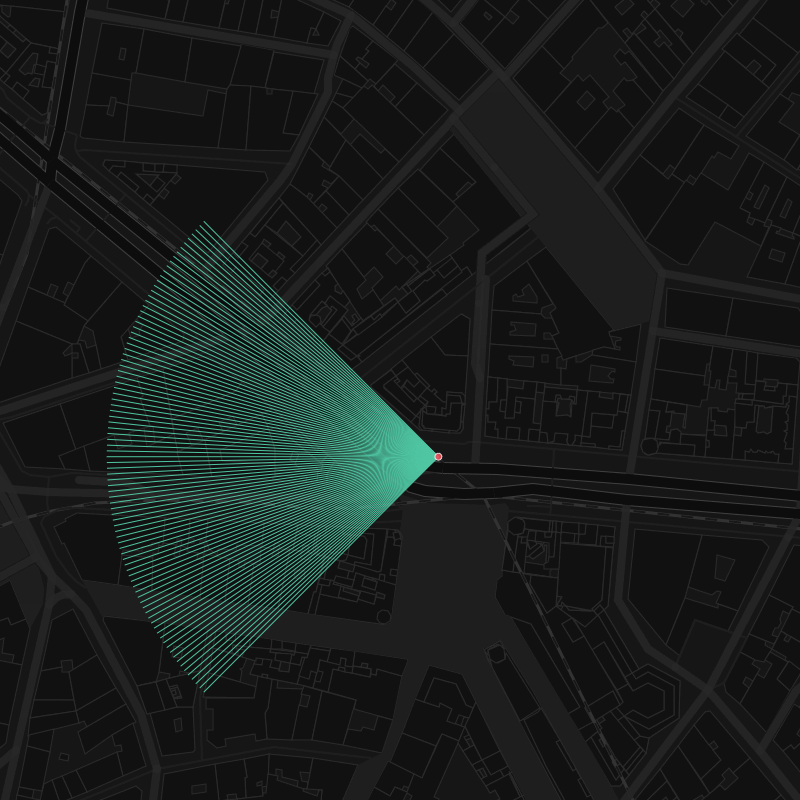5. Now, getting the intersections between the rays and the polygons:

``````    intersections AS(
SELECT
r.id,
(ST_Dump(
ST_Intersection(
-- to avoid intersections with polygon holes
ST_Boundary(b.geom),
r.geom
)
)).geom AS point
FROM
rays r
LEFT JOIN
buildings b
ON
ST_Intersects(b.geom,r.geom)
),
``````

So we translated our rays spectrum into a cloud of points of the intersections between all the rays and all the (external boundaries of the) polygons within range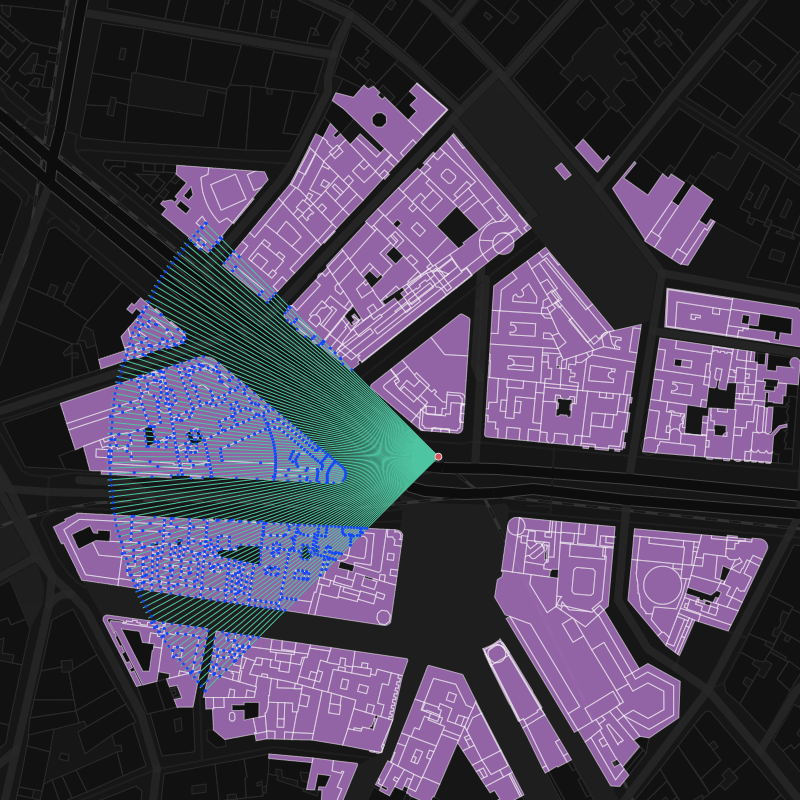6. Now, we need to rank the intersections in terms of distance to `center`

``````    intersections_distances AS(
SELECT
id,
point as geom,
row_number() over(partition by id order by center <-> point) as ranking
FROM intersections
),
``````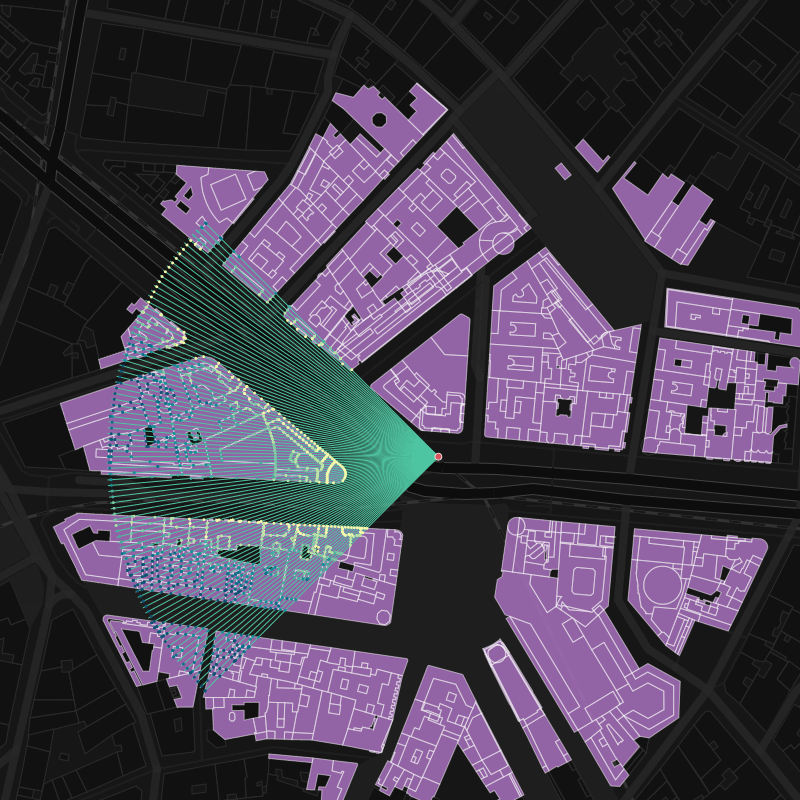7. …in order to keep only the closest intersection per polygon

``````    intersection_closest AS(
SELECT
-1 as id,
CASE WHEN fov = 360 THEN null::geometry ELSE center END as geom
UNION ALL
(SELECT
id,
geom
FROM intersections_distances
WHERE ranking = 1
ORDER BY ID)
UNION ALL
SELECT
999999 as id,
CASE WHEN fov = 360 THEN null::geometry ELSE center END as geom
),
``````

We are adding the center point twice (before and after all the intersections) in case the `fov` is less than 360º…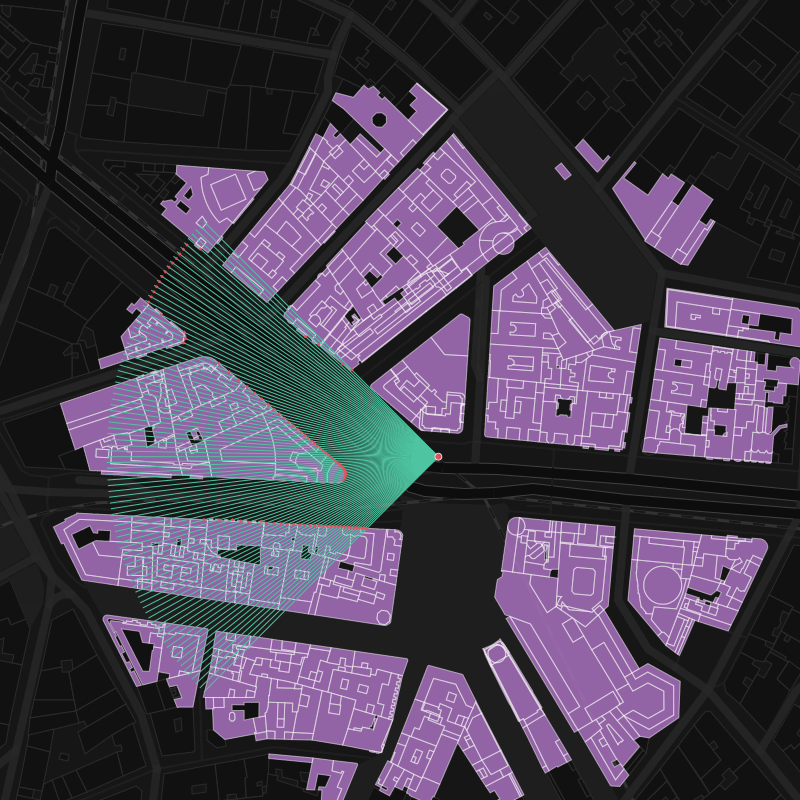8. … so we can close the line and build a polygon (the isovist!) with it

``````    isovist_0 AS(
SELECT
ST_MakePolygon(ST_MakeLine(geom)) as geom
FROM intersection_closest
),
``````

9. Let’s get the `polygons` that actually intersects my isovist

``````    isovist_buildings AS(
SELECT
-- avoid geometry collections
ST_CollectionExtract(ST_union(b.geom),3) as geom
FROM
isovist_0 i,
buildings_crop b
WHERE ST_Intersects(b.geom,i.geom)
)
``````

10. Because of the `arc` resolution, we need to crop the resulting isovist with the `polygons` above to have the best level of detail. Because of this PostGIS ticket we need to sanitize the results for the cases there’s no building intersecting my isovist (all of them are further than the horizon), and it’s a plain circular arc.

``````       SELECT
coalesce(ST_Difference(i.geom, b.geom), i.geom) into geomout
FROM
isovist_0 i,
isovist_buildings b;
``````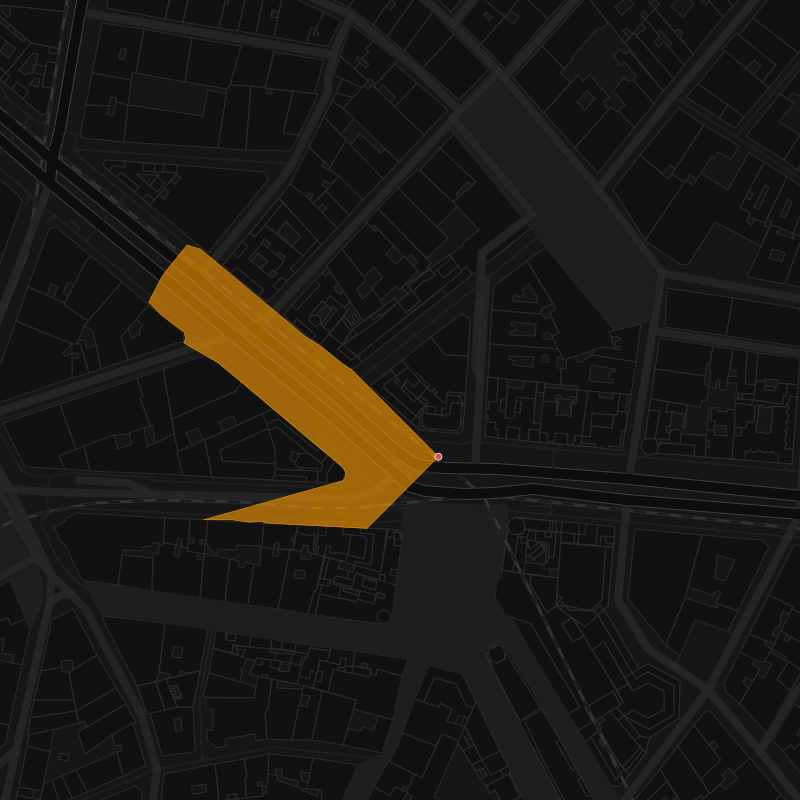So, finally, the full function should look like:

``````CREATE OR REPLACE FUNCTION ISOVIST(
IN center geometry,
IN polygons geometry[],
IN rays integer DEFAULT 36,
IN fov integer DEFAULT 360
)
RETURNS geometry  AS \$\$
DECLARE
arc numeric;
angle_0 numeric;
geomout geometry;
BEGIN
arc := fov::numerics / rays::numeric;
IF fov = 360 THEN
angle_0 := 0;
ELSE
angle_0 := heading - 0.5 * fov;
END IF;

WITH
buildings_0 AS(
SELECT
t.geom
FROM unnest(polygons) as t(geom)
),
buildings_crop AS(
SELECT
geom
FROM buildings_0
),
buildings AS(
SELECT geom FROM buildings_crop
UNION ALL
),
rays AS(
SELECT
t.n as id,
ST_SetSRID(
ST_MakeLine(
center,
ST_Project(
center::geography,
)::geometry
),
4326) AS geom
FROM generate_series(0, rays) as t(n)
),
intersections AS(
SELECT
r.id,
(ST_Dump(ST_Intersection(ST_Boundary(b.geom),r.geom))).geom AS point
FROM
rays r
LEFT JOIN
buildings b
ON
ST_Intersects(b.geom,r.geom)
),
intersections_distances AS(
SELECT
id,
point as geom,
row_number() over(partition by id order by center <-> point) as ranking
FROM intersections
),
intersection_closest AS(
SELECT
-1 as id,
CASE WHEN fov = 360 THEN null::geometry ELSE center END as geom
UNION ALL
(SELECT
id,
geom
FROM intersections_distances
WHERE ranking = 1
ORDER BY ID)
UNION ALL
SELECT
999999 as id,
CASE WHEN fov = 360 THEN null::geometry  ELSE center END as geom
),
isovist_0 AS(
SELECT
ST_MakePolygon(ST_MakeLine(geom)) as geom
FROM intersection_closest
),
isovist_buildings AS(
SELECT
ST_CollectionExtract(ST_union(b.geom),3) as geom
FROM
isovist_0 i,
buildings_crop b
WHERE ST_Intersects(b.geom,i.geom)
)
SELECT
coalesce(ST_Difference(i.geom, b.geom), i.geom) into geomout
FROM
isovist_0 i,
isovist_buildings b;

RETURN geomout;
END;
\$\$ language plpgsql IMMUTABLE;
``````

Magic!

Tags:

Categories:

Updated: